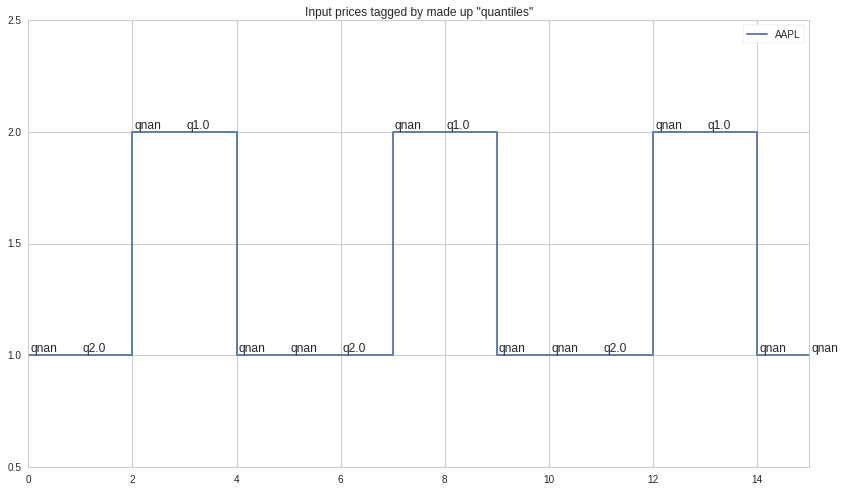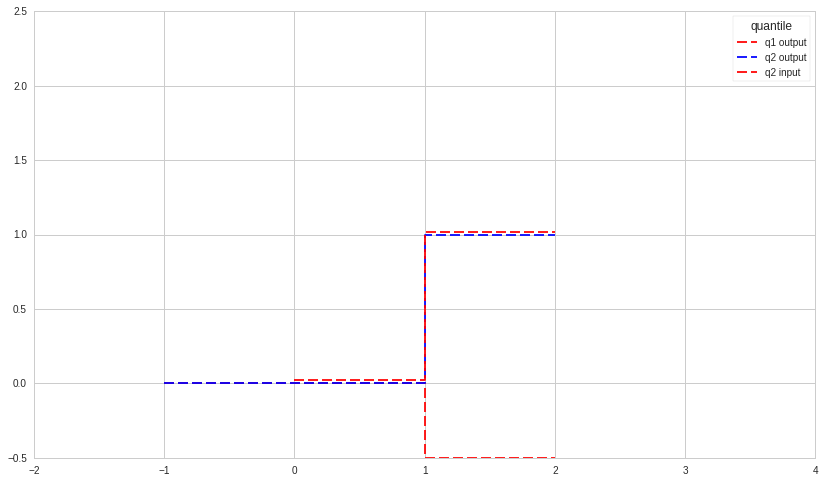Notebook
In :
import pandas as pd
import alphalens
import matplotlib as mpl
import matplotlib.pyplot as plt
import numpy as np

factor_pattern = [1.,2.,2.] # compute cumulative performance of this repeated pattern
padding_periods = 2 # how many periods to put between pattern repetitions
pattern_count = 3 # how may times to repeat pattern
prices = [1.] + (factor_pattern + [1.]*padding_periods)*pattern_count
prices = pd.DataFrame({'date':pd.date_range('2016-01-01', periods=len(prices)),'AAPL':prices}).set_index('date')
factors = prices.stack()
factors.loc[:] = [np.nan, 2, np.nan, 1, np.nan, np.nan, 2, np.nan, 1, np.nan, np.nan, 2, np.nan, 1,np.nan, np.nan]
factors.index.set_names('asset', 1, True)
print ' prices', prices.AAPL.values.tolist(), '\nfactors', ','.join(factors.map('   {0}'.format)),\
'\nquantile 1 is padding, quantile 2 is the tested signal'

prices.reset_index().plot(linestyle='steps-post', title='Input prices tagged by made up "quantiles"')
plt.ylim(0.5, max(factor_pattern)+.5)
for bar, factor in factors.reset_index().iterrows():
#     print factor
plt.annotate('q%s'%factor, (bar,prices.iloc[bar]),(2,4), textcoords='offset points')
# plt.title()

 prices [1.0, 1.0, 2.0, 2.0, 1.0, 1.0, 1.0, 2.0, 2.0, 1.0, 1.0, 1.0, 2.0, 2.0, 1.0, 1.0]
factors    nan,   2.0,   nan,   1.0,   nan,   nan,   2.0,   nan,   1.0,   nan,   nan,   2.0,   nan,   1.0,   nan,   nan
quantile 1 is padding, quantile 2 is the tested signalIn :
cumulret = alphalens.performance.average_cumulative_return_by_quantile(factors, prices, 1, 2, demeaned=False)
cumulret.index.set_names(['quantile', 'moment'], inplace=True)
print 'average_cumulative_return_by_quantile:\n', cumulret
cumulret = cumulret.loc[(slice(None),'mean'),:].reset_index(1,True)
# print cumulret
cumul_plot = cumulret[1:].T.rename_axis({1: 'q1 output', 2:'q2 output'},1)
visual_offset = 0.02
cumul_plot['q2 input'] = prices.reset_index()[1:6]['AAPL'].reset_index(drop=True)-1+visual_offset
cumul_plot.plot(linestyle='steps-post', dashes=(10,4), color=['r', 'b'])
print """The output signal 'quantile 2' is very different from the input not only in scale but in direction.
For a single repeated pattern the output could be the same as the input if the input is at least as long
as the requested forward period. If the input instance is shorter then it is acceptable to use other
following quantiles. The current output is convoluted using its own parts and also it's neighbors
(different quantiles). The standard deviation is also incorrect (too high, but actually 0 b/c we have a
single repeating pattern in q2)
"""
plt.ylim(-.5,2.5)
plt.xlim(-2, 4);

average_cumulative_return_by_quantile:
-1    0    1    2
quantile moment
1.0      mean    0.0  0.0 -0.5 -0.5
std     0.0  0.0  0.0  0.0
2.0      mean    0.0  0.0  1.0  1.0
std     0.0  0.0  0.0  0.0
The output signal 'quantile 2' is very different from the input not only in scale but in direction.
For a single repeated pattern the output could be the same as the input if the input is at least as long
as the requested forward period. If the input instance is shorter then it is acceptable to use other
following quantiles. The current output is convoluted using its own parts and also it's neighbors
(different quantiles). The standard deviation is also incorrect (too high, but actually 0 b/c we have a
single repeating pattern in q2)